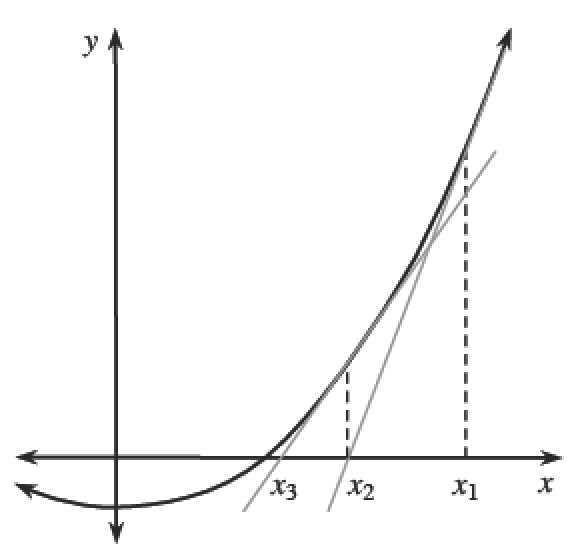### Home > APCALC > Chapter 8 > Lesson 8.4.1 > Problem8-141

8-141.

Without looking at the graph, use Newton’s Method to approximate the roots of $f ( x ) = \frac { 1 } { 1 + x ^ { 2 } }$ if $x_1 = 1$. What happened?

What happened?
Think about the shape of the graph of $f(x)$.
What is its range? What does that mean about the roots?

##### Newton's Method for Approximating Roots

Newton’s Method uses an iterative process to approximate a root ($x$-intercept) of a function.

$⇒$Begin with a point $x_{1}$ near the root. Write the equation of its tangent line at $x_{1}: y – f\left(x_{1}\right) = f ′\left(x_{1}\right)\left(x – x_{1}\right)$.

The $x$-intercept of this tangent line is $x_{2}$.
Solve for $x_{2}$ by setting $y = 0$.

$0 − f\left(x_{1}\right) = f ′\left(x_{1}\right)\left(x_{2} − x_{1}\right),$ so $x _ { 2 } = x _ { 1 } - \frac { f ( x _ { 1 } ) } { f ^ { \prime } ( x _ { 1 } ) }$.

$⇒$ To calculate $x_{3}$, solve for the root of the line tangent to $f$ at $x_{2}$. This process continues until the desired accuracy is met.

Therefore, each new approximation, $x_{n+1}$, can be found using $x _ { n + 1 } = x _ { n } - \frac { f ( x _ { n } ) } { f ^ { \prime } ( x _ { n } ) }$. Newton’s Method was very important before calculators were invented and represents an excellent example of an iterative process. For most functions it works well, but it is always possible to find examples where it does not.